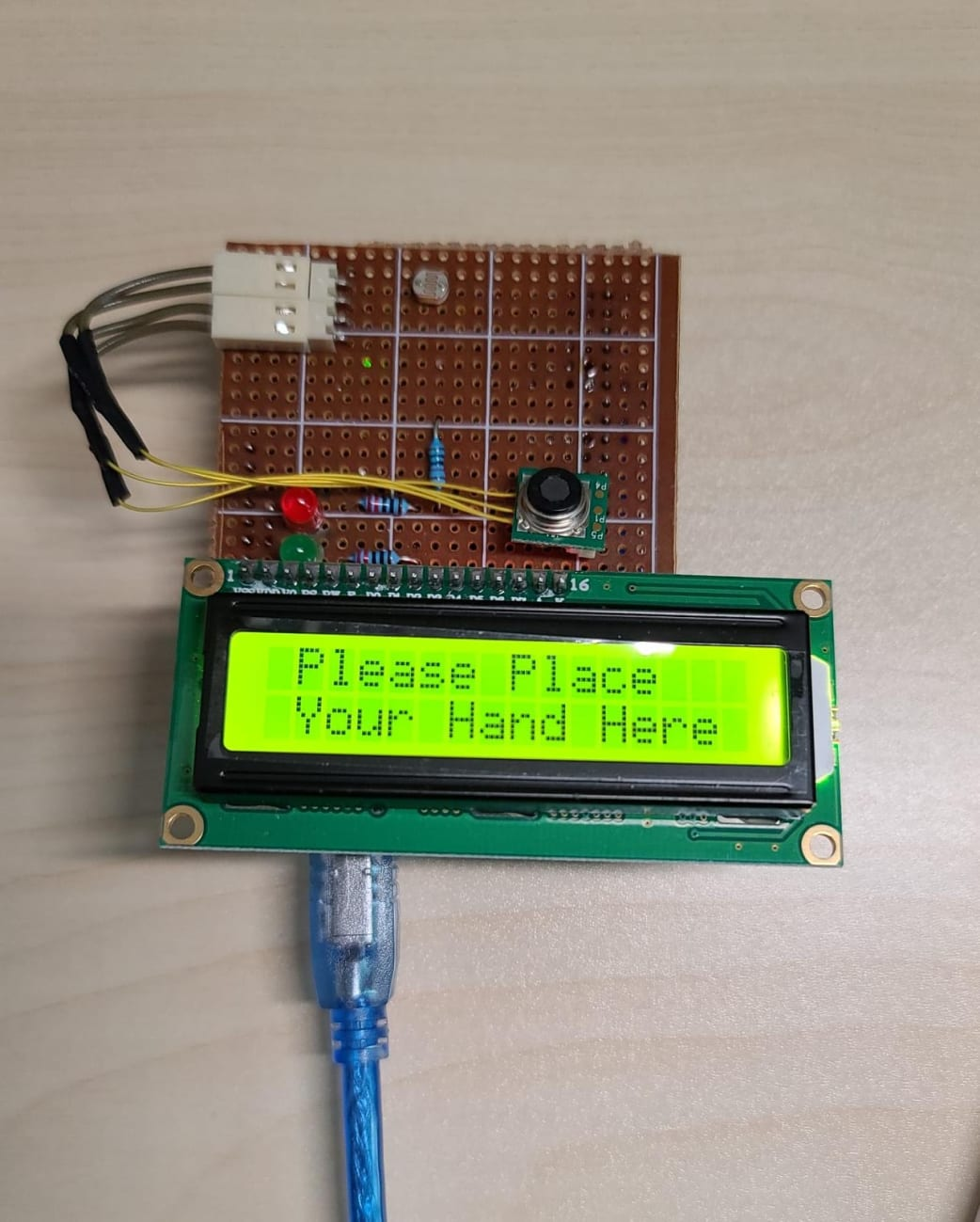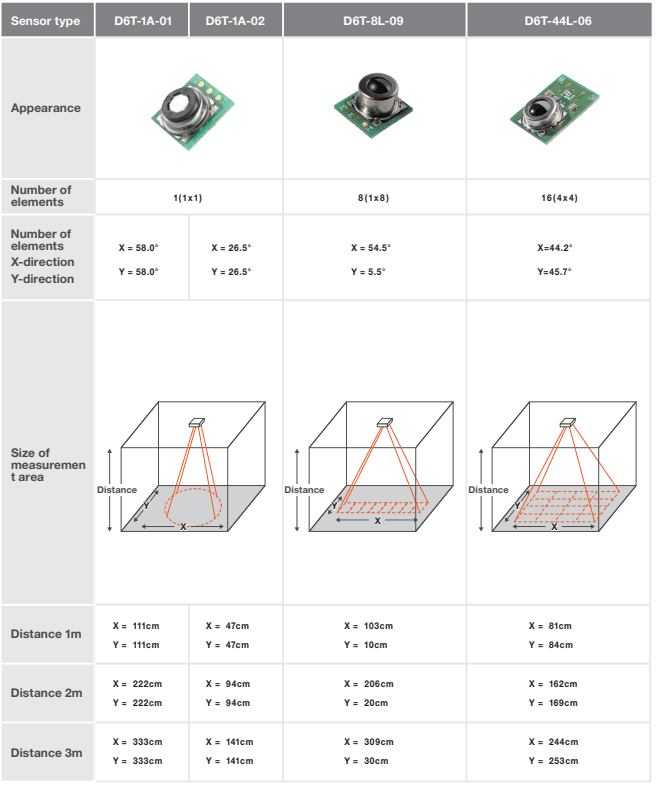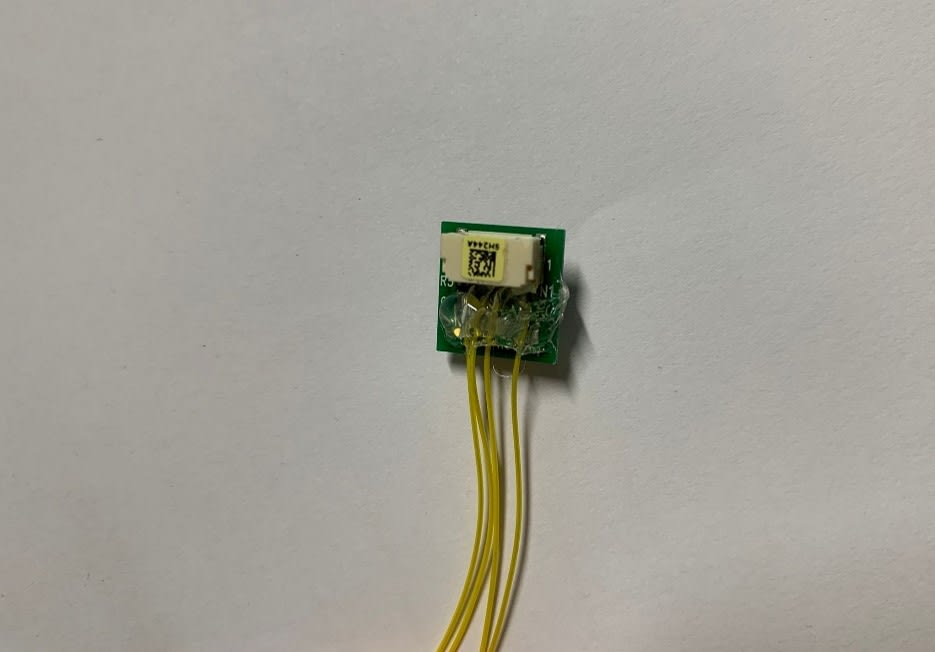The COVID-19 situation in Hong Kong has gotten worse recently, and I don't have a thermometer at home. There are many different types of thermometers on the market, and some are very cheap. But I don't think most of them are accurate, and if I buy expensive ones, I'd better make them myself. Therefore, I bought an Omron thermal sensor D6T-1A-02 to try and make a thermometer myself.

D6T-1A-02 is a thermal sensor under the Omron D6T series thermal sensor. This is a 1x1 size measurement area, and the viewing angle is 26.5°, 26.5° (X direction, Y direction). The storage temperature range is -40 to 80°C, and the accuracy is ±1.5°C under Vcc = 5V. The Omron D6T series thermal sensor mainly has three element types of thermal sensor, the power supply voltage both are 4.5 to 5.5 VDC, the difference is the size of measurement, which have 1x1, 1x8, and 4x4. Because I want to make a personal thermometer this time, 1x1 is enough.After I got the D6T-1A-02 sensor, I forgot to buy a GHR-04V-S connecter cable, so I did a silly thing and soldered the cable on the PCB header directly. I don’t recommend you do this, but because of the current COVID-19 situation in Hong Kong, this is my own choice. But it doesn’t matter, and I can finally connect like this,Then now I can start programming the thermal sensor.

D6T-1A-02 has a sample program in GitHub, which is for the Arduino MKR-WiFi1010 / Adafruit Feather ESP32.  I tried to make it for the Arduino UNO, which also worked. But as the sample program keeps measuring the temperature, making it to a personal thermometer still has a distance. So, I rewrote the program and used a photoresistor to trigger it and display the measured temperature on a 16x2 LCD display.

``````#include <Wire.h>
#include <LiquidCrystal_I2C.h>

LiquidCrystal_I2C lcd(0x27, 16, 2);

#define D6T_CMD 0x4C  // for D6T-44L-06/06H, D6T-8L-09/09H, for D6T-1A-01/02

#define N_ROW 1
#define N_PIXEL 1
#define N_READ ((N_PIXEL + 1) * 2 + 1)

#define Red 13
#define Green 12
``````

Here is the setup of the D6T-1A-02 thermal sensor, LCD display and the pin mode of the led button pin.

``````void setup() {
Serial.begin(9600);  // Serial baudrate = 9600bps
Wire.begin();  // i2c master
pinMode(Red, OUTPUT);
pinMode(Green, OUTPUT);
lcd.init();                      // initialize the lcd
lcd.backlight();
delay(220);
}
``````

And here is the setup of the program.

``````void loop() {
int i = 0;
int16_t itemp = 0;
//Serial.println(value);    //Check photoresistor value

digitalWrite(Green, LOW);
digitalWrite(Red, LOW);

lcd.setCursor(1,0);
lcd.setCursor(1,1);

if(value<400){
delay(300);
// I2C buffer of "Arduino MKR" is 256 buffer. (It is enough)
Wire.write(D6T_CMD);               // D6T register
Wire.endTransmission();
while (Wire.available()) {
}

//Convert to temperature data (degC)
ptat = (double)conv8us_s16_le(rbuf, 0) / 10.0;
for (i = 0; i < N_PIXEL; i++) {
itemp = conv8us_s16_le(rbuf, 2 + 2*i);
pix_data[i] = (double)itemp / 10.0;
}
Displaytemp();

}
delay(100);
}
``````

Here is the main function of the program.

I used “int value = analogRead(A0);” to check the photoresistor’s value, which should go from max and min values of 0 (0V) to 1023(5V).

In the normal situation, the LCD Display will show “Please Place Your Hand Here”, as the columns are limited, I was pleased it as two rows.

Then I checked in my scenario, if the photoresistor value is around 800, and if I place my hand on the photoresistor, the value will drop to around 300. Therefore, I place an if-loop to check whether the photoresistor value is small than 400 to trigger the thermometer.

Also, there has a function named “Displaytemp()”, which is used to display the measured temperature and use the LED to check whether you are fever or not.

``````void Displaytemp(){
int i = 0;
for (i = 0; i < N_PIXEL; i++) {
Serial.println(pix_data[i], 1);
if((pix_data[i])<30){
lcd.clear();
digitalWrite(Green, HIGH);
digitalWrite(Red, HIGH);
lcd.setCursor(1,0);
lcd.print("YourTemperature is:");
lcd.setCursor(1,1);
lcd.print((pix_data[i]));
}
else if ((pix_data[i])>=30 && (pix_data[i])<=37){
lcd.clear();
digitalWrite(Green, HIGH);
digitalWrite(Red, LOW);
lcd.setCursor(1,0);
lcd.print("YourTemperature:");
lcd.setCursor(1,1);
lcd.print((pix_data[i]));
}
else if((pix_data[i])>37){
lcd.clear();
digitalWrite(Green, LOW);
digitalWrite(Red, HIGH);
lcd.setCursor(1,0);
lcd.print("YourTemperature:");
lcd.setCursor(1,1);
lcd.print((pix_data[i]));
}
else{
lcd.clear();
digitalWrite(Green, LOW);
digitalWrite(Red, LOW);
lcd.setCursor(1,0);
lcd.print("YourTemperature:");
lcd.setCursor(1,1);
lcd.print((pix_data[i]));
}
delay(3000);
lcd.clear();
}
}
``````

Here is also some function to trigger the thermometer.

``````uint8_t calc_crc(uint8_t data) {
int index;
uint8_t temp;
for (index = 0; index < 8; index++) {
temp = data;
data <<= 1;
if (temp & 0x80) {data ^= 0x07;}
}
return data;
}

bool D6T_checkPEC(uint8_t buf[], int n) {
int i;
for (i = 0; i < n; i++) {
crc = calc_crc(buf[i] ^ crc);
}
bool ret = crc != buf[n];
if (ret) {
Serial.print("PEC check failed:");
Serial.print(crc, HEX);
Serial.print("(cal) vs ");
Serial.print(buf[n], HEX);
Serial.println("(get)");
}
return ret;
}

int16_t conv8us_s16_le(uint8_t* buf, int n) {
uint16_t ret;
ret = (uint16_t)buf[n];
ret += ((uint16_t)buf[n + 1]) << 8;
return (int16_t)ret;   // and convert negative.
}
``````

Great! Now I have managed to have a thermometer of my own design at home.

Hope the covid 19 will end soon! And the thermometer becomes unnecessary in our daily life.

JulianWong has not written a bio yet…